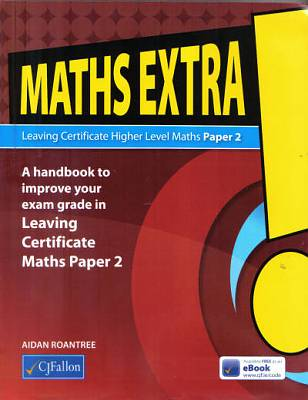# Maths Extra! Leaving Certificate Higher Level Maths Paper 2

Welcome to Maths Extra! Higher Level Paper 2. This book is a concise, exam-focused handbook for the Leaving Certificate Maths, Paper 2 exam. It contains all the information you will need in your preparation for this exam.

You can use Maths Extra! Higher Level Paper 2 in conjunction with your textbook, as you make your way through the maths course. It will help you to read, plan, time and answer your exam questions in the most efficient and effective manner.

This book summarises all the key concepts, formulae and methods in each of the topic areas that is covered by Paper 2. They are presented in an accessible, clear format where much of the long backgrounds, already covered in your textbook, has been omitted or briefly covered.

This revision book is divided into two sections. The first twelve chapters cover Strand 2, Geometry and Trigonometry, along with part of Strand 3 devoted to areas and volumes. In these chapters, we start with geometry, because many of the ideas from geometry, as well as being examinable in their own right, are frequently referred to, or required, in questions which are seen as being primarily trigonometry or co-ordinate geometry questions. Much and all as many students do not take to geometry, they need to realise its importance for many other topics.

The last eight chapters cover Strand 1, Probability and Statistics. This strand can really be divided into three sections: pure probability, pure statistics and probability and statistics, where ideas from both topics are used together to tackle problems in inferential statistics.

Chapters 13 to 15 cover pure probability, its concepts, formula and its applications. As very few of the required formulae in this section are in the Formulae and Tables, it is important that you learn all the relevant formulae, as well as being able to use them.

Chapters 16 to 18 deal with pure statistics. First of all is the idea of learning how to gather reliable data, not just any data. Then comes the process of presenting the data graphically and analysing it to be able to describe the data in a mathematical way. In Chapter 18, the idea of correlation is investigated for paired data.

In the final two chapters, probability and statistics are combined to deal with normal distributions and use them to express confidence in results obtained empirically.

ISBN: 9780714423838

€ 11.30

In Stock.

Usually despatched in 2-5 working days.Paperback

Maths Extra! Leaving Certificate Higher Level Maths Paper 2 also appears in these Categories: